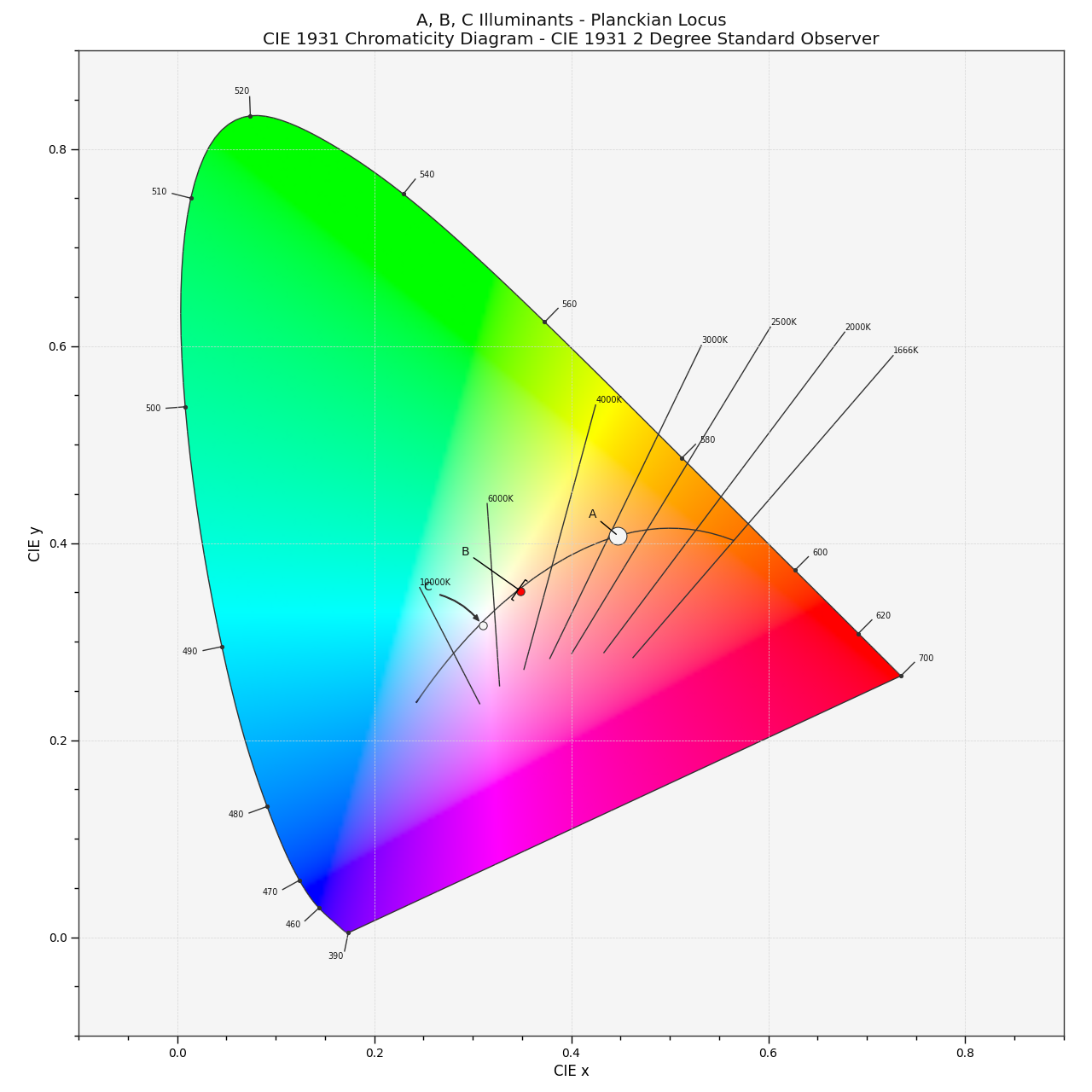# colour.plotting.temperature.plot_planckian_locus_in_chromaticity_diagram¶

colour.plotting.temperature.plot_planckian_locus_in_chromaticity_diagram(illuminants=None, annotate_parameters=None, chromaticity_diagram_callable=<function plot_chromaticity_diagram>, planckian_locus_callable=<function plot_planckian_locus>, method='CIE 1931', **kwargs)[source]

Plots the Planckian Locus and given illuminants in the Chromaticity Diagram according to given method.

Parameters
• illuminants (array_like, optional) – Factory illuminants to plot.

• annotate_parameters (dict or array_like, optional) – Parameters for the plt.annotate() definition, used to annotate the resulting chromaticity coordinates with their respective illuminant names if annotate is set to True. annotate_parameters can be either a single dictionary applied to all the arrows with same settings or a sequence of dictionaries with different settings for each illuminant.

• chromaticity_diagram_callable (callable, optional) – Callable responsible for drawing the Chromaticity Diagram.

• planckian_locus_callable (callable, optional) – Callable responsible for drawing the Planckian Locus.

• method (unicode, optional) – {‘CIE 1931’, ‘CIE 1960 UCS’, ‘CIE 1976 UCS’}, Chromaticity Diagram method.

Other Parameters

**kwargs (dict, optional) – {colour.plotting.artist(), colour.plotting.diagrams.plot_chromaticity_diagram(), colour.plotting.temperature.plot_planckian_locus(), colour.plotting.render()}, Please refer to the documentation of the previously listed definitions.

Returns

Current figure and axes.

Return type

tuple

Examples

>>> plot_planckian_locus_in_chromaticity_diagram(['A', 'B', 'C'])
...
(<Figure size ... with 1 Axes>, <matplotlib.axes._subplots.AxesSubplot object at 0x...>)